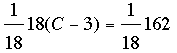SEARCH HOMEMath Central Quandaries & QueriesQuestion from Alicia, a student: 18(C-3)=162 adult student returning to school after 20 years and then never had it in school. Just doesn't click yetHi Alicia,

You want to start with the equation

18(C-3) = 162,

manipulate it through a number of steps and end with an equation of the form

C = some number.

The changes you make at each step must maintain the equality of the two sides of the equation. The operations that will guarantee this are adding the same amount to each side and multiplying each side by the same amount.

Here is one way to approach your problem.

To eliminate the 18 you can multiply each side by 1/18. This givesOn the left side 18 divided by 18 is 1 and on the right side 162/18 = 9 so the equation becomes

C - 3 = 9.

Finally adding 3 to each side yields

C - 3 + 3 = 9 + 3

or

C = 12.

A second way to approach 18(C-3) = 162 is to first expand the left side. This gives

18C - 18 × 3 = 162

or

18C - 54 = 162.

Now add 54 to each side

18C - 54 + 54 = 162 + 54

or

18C = 216.

At this stage multiply each side by 1/18 to get

C = 216/18 = 12.

I hope this helps,
PennyMath Central is supported by the University of Regina and The Pacific Institute for the Mathematical Sciences.